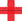top of page

## Video ResourcesIn this video, Luke or Lindsey takes students through a full Stats Medic EFFL lesson.

# Sampling Distribution for the Difference Between Two Proportions

##### Learning Targets
• Calculate the mean and standard deviation of the sampling distribution of a difference between sample proportions.

• If appropriate, use a Normal distribution to calculate probabilities involving a difference between two proportions.

bottom of page